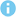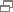Current Position：HOMESeven Bank Account HoldersDeposits and LoansLoan ServicesInterest

# Loan Service: Interest

The loan interest differs as follows depending on the borrowing limit.

Borrowing limit—100,000 yen, 300,000 yen or 500,000 yen:
15% per annum (applicable as of August 17, 2020, and onward; subject to change)

Borrowing limit—700,000 yen or 1,000,000 yen:
14% per annum (applicable as of August 17, 2020, and onward; subject to change)

## The daily interest rate is calculated based on addition of interest to principal.

### Daily interest rate calculation

The daily interest rate is calculated based on the following formula (1 year is calculated as 365 days).
The interest incurs based on the number of days between the day of the borrowing transaction and the day before the repayment date.

Outstanding borrowing amount × Borrowing interest rate × Number of days elapsed / 365 days ＝ Amount of interest that incurs

•Minimum unit of money that incurs interest is 100 yen (Outstanding borrowing amount is divided into units used for calculating interest). Any amount that is under 100 yen is rounded down.
(Ex: 77,777 yen ⇒ 77,700 yen)

•Any interest that is under 1 yen is rounded down.
(Ex: 46.575…yen ⇒ 46 yen)

Specific examples of daily interest rate calculation### Addition of interest to principal

#### ■What is addition of interest to principal?

The addition of interest to principal refers to the incurred interest being included in the outstanding borrowing amount. The interest that incurs between the previous monthly repayment date, or the initial borrowing date, and the current monthly repayment date is calculated and included in the outstanding borrowing amount. This is referred to as the addition of interest to principal.
The addition of interest to principal is added into the outstanding borrowing amount, and the monthly repayment amount for the outstanding borrowing amount is due on the monthly repayment date.

Specific examples of addition of interest to principal#### ■Processing of addition of interest to principal when monthly repayment is past due

In the event that the monthly repayment is past due, be aware that the interest incurs on the total outstanding borrowing amount (which includes the addition of interest to principal).

Specific examples of processing when monthly repayment is past due